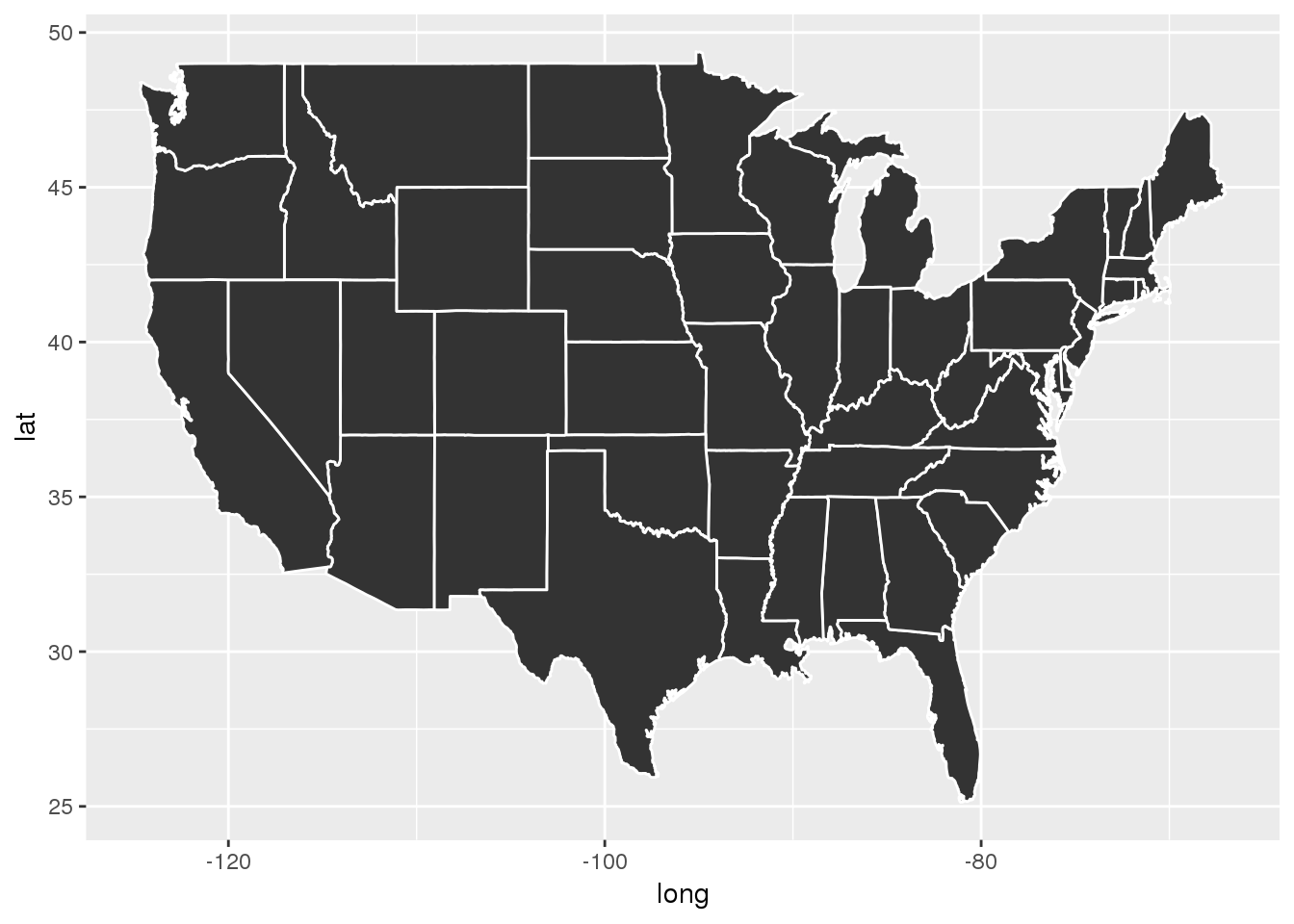If you shut down R, you will need to change your working directory and run the following commands to load libraries,

``````library('ggplot2')
library('dplyr')
library('tidyr')
library('ISDSWorkshop')
workshop(launch_index=FALSE)``````

Read in the data files and create additional variables

``````# Read csv files and create additional variables
GI     = read.csv("GI.csv") %>%
mutate(
date      = as.Date(date),
weekC     = cut(date, breaks="weeks"),
week      = as.numeric(weekC),
weekD     = as.Date(weekC),
facility  = as.factor(facility),
icd9class = factor(cut(icd9,
breaks = icd9df\$code_cutpoint,
labels = icd9df\$classification[-nrow(icd9df)],
right  = TRUE)),
ageC      = cut(age,
breaks = c(-Inf, 5, 18, 45 ,60, Inf)),
zip3      = trunc(zipcode/100))``````

## Exporting tables

There are many ways to export tables (for use in Word). Here I will cover two basic approachess that are simple and work well.

• cut-and-paste
• create HTML table and cut-and-paste to Word

There are more sophisticated approaches that I will not cover:

### Cut-and-paste

You can cut-and-paste directly from R.

``````ga_l <- GI %>%
group_by(gender, ageC) %>%
summarize(count = n())

ga_w <- ga_l %>%
print(row.names = FALSE)``````
``````## Source: local data frame [2 x 6]
## Groups: gender 
##
##   gender `(-Inf,5]` `(5,18]` `(18,45]` `(45,60]` `(60, Inf]`
## * <fctr>      <int>    <int>     <int>     <int>       <int>
## 1 Female       2161     1942      3710      1590        1250
## 2   Male       2163     1860      3766      1543        1259``````

Cut-and-pasting this table is done in ASCII format. This looks good in a plain text document, e.g. Notepad, TextEdit, and some email, but will not look good in other formats, e.g. docx.

### Create HTML table

``````library('xtable')
tab = xtable(ga_w,
caption = "Total GI cases by Sex and Age Category",
label   = "myHTMLanchor",
align   = "ll|rrrrr") # rownames gets a column``````

Save the table to a file

``print(tab, file="table.html", type="html", include.rownames=FALSE)``

### Output for this HTML table

The HTML code looks like

``print(tab, type="html", include.rownames=FALSE)``
``````## <!-- html table generated in R 3.3.1 by xtable 1.8-2 package -->
## <!-- Thu Jan 19 12:50:30 2017 -->
## <table border=1>
## <caption align="bottom"> Total GI cases by Sex and Age Category </caption>
## <tr> <th> gender </th> <th> (-Inf,5] </th> <th> (5,18] </th> <th> (18,45] </th> <th> (45,60] </th> <th> (60, Inf] </th>  </tr>
##   <tr> <td> Female </td> <td align="right"> 2161 </td> <td align="right"> 1942 </td> <td align="right"> 3710 </td> <td align="right"> 1590 </td> <td align="right"> 1250 </td> </tr>
##   <tr> <td> Male </td> <td align="right"> 2163 </td> <td align="right"> 1860 </td> <td align="right"> 3766 </td> <td align="right"> 1543 </td> <td align="right"> 1259 </td> </tr>
##    <a name=myHTMLanchor></a>
## </table>``````

and the resulting table looks like

``print(tab, type="html", include.rownames=FALSE)``
Total GI cases by Sex and Age Category
gender (-Inf,5] (5,18] (18,45] (45,60] (60, Inf]
Female 2161 1942 3710 1590 1250
Male 2163 1860 3766 1543 1259

### Copy-and-paste table to Word

Now you can

1. Open the file (`table.html`)
2. Copy-and-paste this table to Word.

### Activity - exporting tables

Create a Word table for the number of cases by facility and age category.

``````# Summarize data by facility and age category

# Reshape data from long to wide format

# Create HTML table

# Save HTML to file

# Copy-and-paste table into Word``````

When you have completed the activity, compare your results to the solutions.

## Maps

The packages `ggplot2` and `maps` can be used together to make maps.

Map of the continental US

``````library('maps')
states = map_data("state")
ggplot(states, aes(x = long, y = lat, group = group)) +
geom_polygon(color="white")``````Map of the counties in the continental US

``````counties = map_data("county")
ggplot(counties, aes(x = long, y = lat, group = group)) +
geom_polygon(color = "white")``````